# Reading Numbers in the Thousands• We want to read the large number 867 485 using a place value chart.
• We group the into groups of three. We have the thousands group and then the hundreds, tens and units group.
• We break the thousands group into hundreds, tens and units. We have 867.
• So we say we have eight hundred and sixty-seven thousand.
• In the hundreds, tens and units columns we have 485, which is four hundred and eighty-five.
• We have eight hundred and sixty-seven thousand, four hundred and eighty-five.
We read the thousands group as hundreds tens and units, followed by a ‘thousand’.

We then read the hundreds, tens and units, putting a comma first if we have a digit in the hundreds column.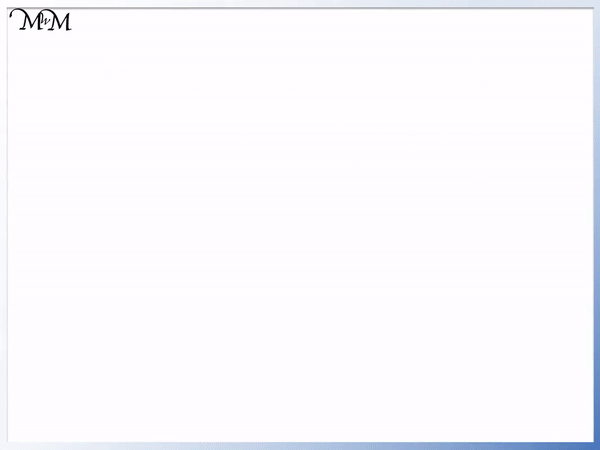• We want to read the large number 746 002.
• It has 6 , which we separate into groups of 3 on our place value chart.
• Reading the thousands we have, seven hundred and forty-six.
• Reading the hundreds, tens and units, we just have two.
• Our number is seven hundred and forty-six thousand and two.Supporting Lessons# How to Read Numbers in the Thousands using a Thousands Place Value Chart

To read numbers in the thousands we put the digits into their place value columns and read from left to right in groups of hundreds, tens and units (ones). We read the hundreds, tens and units within the thousands group and then read the hundreds, tens and units place value columns afterwards.

In this lesson we will look at reading and writing large numbers with up to and including six digits with examples written on a thousands place value chart.

If you are confident in reading and writing three-digit numbers, then you will be able to read any large number.

Here is an example of reading a thousand place value chart:In the above example, we have a six-digit number.

To make this large number easier to read, we put the digits into groups of three, starting from the right.We put the digits into groups of three by using a space in between the groups.

The group of three digits on the left is our thousands group. This is how many thousands we have.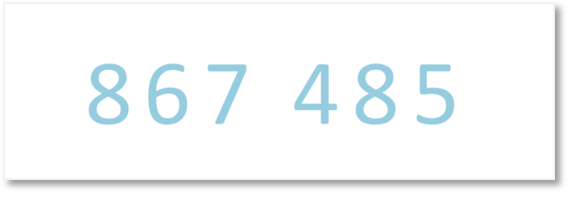Now, that we have put the digits into place value groups of three, we can read the large number.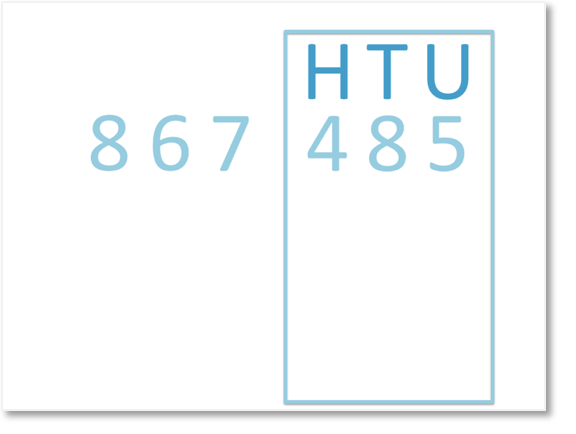The group of digits on the right-hand-side is the hundreds, tens and units.The group of three digits on the left-hand-side is the thousands.We read from left to right, so we start with the thousands place value group on our chart.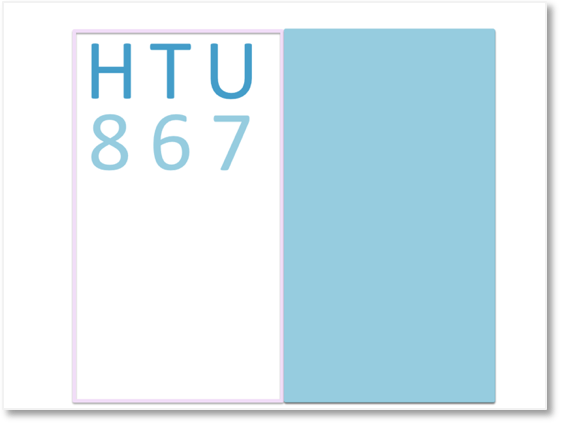We can think of the digits in this group as hundreds, tens and units.

We have eight hundred and sixty-seven.Because these three digits are in the thousands group of our place value chart, we have eight hundred and sixty-seven thousand.Finally, we can read the hundreds, tens and units group.

We have four hundred and eighty-five.

So, the large number is read as:

Eight hundred and sixty-seven thousand, four hundred and eighty-five.

Here is another example of reading a number in the thousands:We begin by putting the digits into groups of three, starting from the right.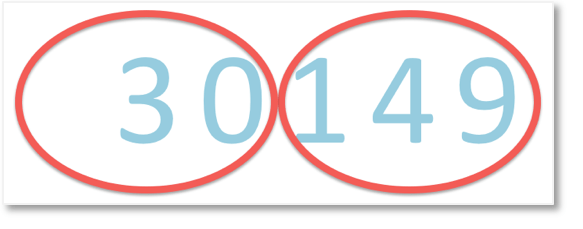We separate these place value groups with a space.Now that we have put the digits into groups of three, we can read the large number.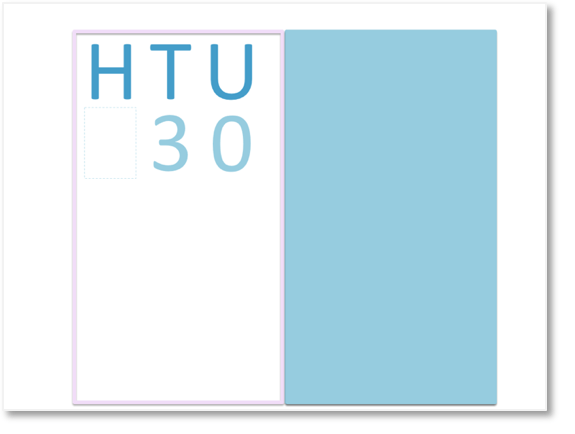We can start by thinking of this place value group as hundreds, tens and units.

We have 30.The digits are in the thousands place value group, so we have thirty thousand.Finally, we read the digits in the hundreds, tens and units place value group of our chart.

We have one hundred and forty-nine.

So, the large number is read as:

Thirty thousand, one hundred and forty-nine.Now try our lesson on Reading Large Numbers in Millions and Billions where we learn how to read larger numbers in the millions and billions using a place value chart.